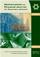Mathematical and Physical Journal
for High Schools
Issued by the MATFUND Foundation
 Already signed up? New to KöMaL?# Contents

Zoltán Bogdán: The 11th International Hungarian Mathematics competition

Solution to a problem of the 43rd International Mathematical Olympiad

Attila Varró: Game Theory in the Dream Factory''

Tamás Szamuely: On the Fields Medal

Imre Légrádi: Ordering watermelons

Dániel Arany Competition in Mathematics, 2001/2003

Hungarian National Competition in Mathematics, 2001/2003

Solutions to exercises for practice (651., 662.)

Solutions to B problems (3431., 3464,. 3499., 3511., 3512., 3513., 3516.)

Solutions to advanced problems A (290.)

New exercises for practice - competition C (725-729.)

New problems - competition B (3652-3661.)

New advanced problems - competition A (323-325.)

Solutions to the programming exercises (25., 26., 27.)

Problems in information technology (55-57.)

Imre Légrádi: The centre of gravity of a triangle

Gyula Radnai: Solutions to the problems of the Eötvös Competition of 2003

Solutions of problems for physics P (3476., 3516., 3524., 3540., 3553.)

Solution to measuring problem M (236.)

Problems in physics (M. 244., P. 3632-3641.)